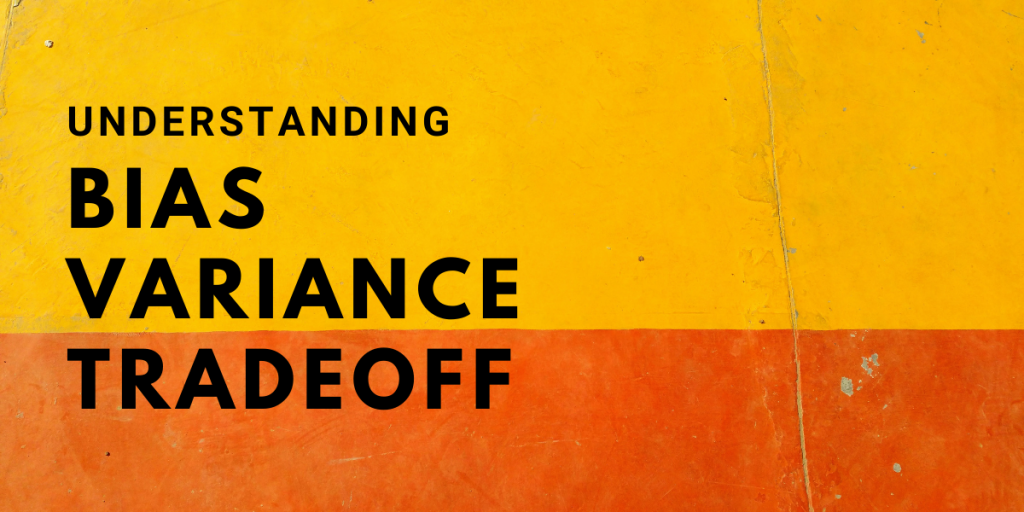# Bias Variance Tradeoff – Understanding the ConceptsTo evaluate a model performance it is essential that we know about prediction errors mainly – bias and variance. Bias Variance tradeoff is a very essential concept in Machine Learning.

Having a Proper understanding of these errors would help to create a good model while avoiding Underfitting and Overfitting the data while training the algorithm.

## What is Bias?

Bias is the difference between the average prediction of our model and the correct target value that the model is trying to predict.

Model having high Bias would oversimply our model and result in more difference in the actual and the predicted value.

To understand Bias let’s look at the figure below:

It is very clear from the figure above that the model or the line did not fit the data well, This is famously termed as Underfitting. This is an example of having High Bias as the difference between the actual value (Blue Data points) and the Predicted values (Red Line) is high.

It always leads to high error on training and test data.

## What is Variance?

Variance is the variability of model prediction for a given data point which tells us spread of our data. So what does high variance looks like?

Models with high variance has a very complex fit to the data, which basically means that our model just memorized the training data. Due to this our model is not able to give correct predictions on the previously unseen data.

such models will perform very well on training data but has high error rates on test data.

This is known as overfitting.

## What is the total error?

Bias and Variance is given by:

• Bias[f'(X)] = E[f'(X) – f(X)]
• Variance[f'(X)] = E[X^2]−E[X]^2

where f(X) is the true value and f'(x) is our model function to predict values close to f(X)

The only important point to notice here is that total error in a model is comprised of three elements.

Total Error = Bias² + Variance + irreducible error

Total error is the sum of Bias², variance and the irreducible error.

Here Irreducible error is the error that can’t be reduced. It is the inherent noise in our data. But we can certainly have control over the amount of Bias and Variance a model can have.

Hence we try to obtain the Optimal values for Bias and Variance by varying the model complexity. we find a good balance between bias and variance such that the total error is minimum.

## Now what is Bias Variance Tradeoff?

If we have a very simple model, this means that we have a high bias, and low variance, as we have seen in the previous section. Similarly, if we get a complex fit on our training data we say that model has high variance and low bias. Either way, we won’t get good results.

So Bias Variance Tradeoff implies that there must be an appropriate balance between model bias and variance so that the total error is minimized without overfitting and underfitting the data.

An optimal balance between bias and variance would never result in overfitting or underfitting.

## Example of Bias Variance Tradeoff in Python

Let’s see how we can calculate bias and variance of a model. run this line on the command prompt to get the package.

```pip install mlxtend
```

You can download the dataset used in this example here (Filename – score.csv).

Let’s see how we can determine the Bias and Variance of a model using mlxtend library.

```#Importing the required modules
from mlxtend.evaluate import bias_variance_decomp
from sklearn.tree import DecisionTreeRegressor
from sklearn.model_selection import train_test_split
import matplotlib.pyplot as plt
from sklearn.metrics import mean_squared_error
import pandas as pd
import numpy as np

x = np.array(df.Hours).reshape(-1,1)
y = np.array(df.Scores).reshape(-1,1)

#Splitting the dataset into train and test set
x_train,x_test, y_train, y_test = train_test_split(x,y, test_size = 0.4 , random_state = 0)

#Making the model
regressor = DecisionTreeRegressor(max_depth = 1)

#Fitting the data to the model
regressor.fit(x_train,y_train)

#Calculating Bias and Variance
avg_expected_loss, avg_bias, avg_var = bias_variance_decomp(
regressor, x_train, y_train, x_test, y_test,
loss='mse',
random_seed=1)

#Plotting the results
x= np.linspace(min(x_train) , max(x_train), 100)
plt.plot(x, regressor.predict(x))
plt.scatter(x_train , y_train , color = 'red')
plt.xlabel('Hours')
plt.ylabel('Score')
plt.title('Model with a High Bias')

print('average Bias: ',avg_bias)
print('average Variance: ',avg_var)

```
```average Bias:  10455.986051700678
average Variance:  61.150793197489904
```